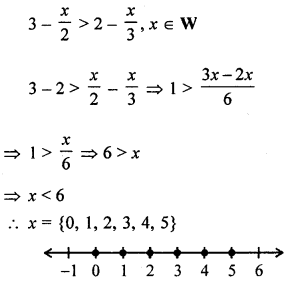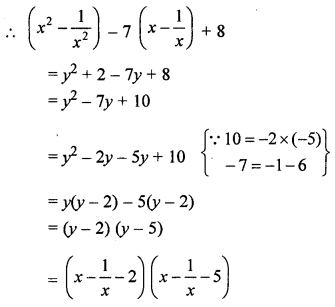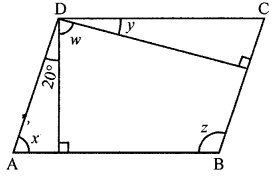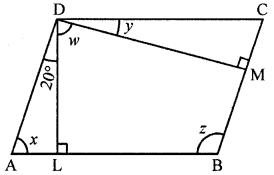## ML Aggarwal Class 8 Solutions for ICSE Maths Model Question Paper 4

Choose the correct answer from the given four options (1-2):
Question 1.
$$\frac{103^{2}-97^{2}}{200}$$ is equal to
(a) 3
(b) 4
(c) 5
(d) 6
Solution:Question 2.
If the’ sum of three consecutive even integers is 36, then the largest integer is
(a) 10
(b) 12
(c) 14
(d) 16
Solution:
Let first number = x
Second number = x + 2
and third = x + 4 x + x + 2 + x + 4 = 36
⇒ 3x + 6 = 36 ⇒ 3x = 36 – 6 = 30
⇒ x= $$\frac{30}{3}$$ = 10
∴ Number are : 10, 10 + 2, 10 + 4
= 10, 12, 14
Largest integer =14 (c)

Question 3.
Find the area of rectangle whose length and breadth are respectively (4x2 – 3x + 7) and (3 – 2x + 3x2).
Solution:
Length of a rectangle (l) = 4x2 – 3x + 7
and breadth of a rectangle (b) = 3 – 2x + 3x2
Area of a rectangle
= (4x2 – 3x + 7) (3 – 2x + 3x2)
= 4x2(3 – 2x + 3x2) – 3x(3 – 2x + 3x2) + 7(3 – 2x + 3x2)
= 12x2 – 8x3 + 12x42 – 9x + 6x2 – 9x3 + 21 – 14x + 21x2
= 12x4 – 17x3 + 39x2 – 23x + 21

Question 4.
Factorize: a2 – c2 – 2ab + b2.
Solution:
a2 – 2ab + b2 – c2
= (a – b)2 – (c)2
= (a – b + c) (a – b – c)

Question 5.
The ages of A and B are in the ratio 3 : 4. Five years later the sum of their ages will be 31 years. What are their present ages?
Solution:
Ratio in the present ages of A and B = 3 : 4
Let A’s age = 3x, then B’s age = 4x
5 years later,
A’s age = 3x + 5 and B’s age = 4x + 5
According to the condition,
3x + 5 + 4x + 5 = 31 7x + 10 = 31
⇒ 7x = 31 – 10 = 21 ⇒ x = $$\frac{21}{7}$$ = 3
∴ A’s present age = 3 × 3 = 9 years
and B’s age = 4 × 3 = 12 years

Question 6.
The sum of the digits of a two digit number is 13. If the number obtained by reversing the digits is 45 more than the original number. Find the original number.
Solution:
Sum of two digits of a 2-digit number = 13
Let unit’s digit = x
Then ten’s digit = 13 – x
and number = x + 10(13 – x)
= x + 130 – 10x
= 130 – 9x …(i)
By reversing the digits,
Unit’s digit =13 – x
and ten’s digits = x
∴ Number = 13 – x + 10x = 13 + 9x
According to the condition,
13 + 9x= 130 – 9x + 45
9x + 9x = 130 – 13 + 45
⇒ 18x= 162
⇒ x= $$\frac{162}{18}$$ = 9
∴ Original number = 130 – 9x
= 130 – 9 × 9 = 130 – 81 =49

Question 7.
The ratio between an exterior angle and interior angle of a regular polygon is 1 : 5, find:
(i) the measure of each exterior angle,
(ii) the measure of each interior angle.
(iii) the number of sides of the polygon.
Solution:
In a regular polygon,
Ratio in exterior angle and interior angle = 1 : 5
Let exterior angle = x
Then interior angle = 5x
but sum of interior angle and exterior angle = 180° (Linear pair)
∴ x + 5x – 180°
⇒ 6x = 30°
∴ x = $$\frac{180^{\circ}}{6}$$ = 30°
(i) ∴ Measure of exterior angle = 30°
(ii) Measure of interior angle = 30° × 5 = 150°
(iii) Number of sidesQuestion 8.
Solve the inequality: 3 – $$\frac{x}{2}$$ > 2 – $$\frac{x}{3}$$ , x ϵ W.
Also represent its solution set on the number line.
Solution:Question 9.
Factorise: x2 + $$\frac{1}{x^{2}}-7\left(x-\frac{1}{x}\right)$$ + 8.
Solution:Question 10.
In the given figure, ABCD is a parallelogram. Find x, y, z and w.Solution:
In ||gm ABCDDL ⊥ AB and DM ⊥ BC
∴ ∠A + ∠L + ∠ADL = 180° (Sum of angles of a triangle)
x + 90° + 20° = 180°
⇒ x + 110° = 180°
x= 180°- 110° = 70°
∠C = ∠A (Opposite angles of a ||gm)
∠C = 70°
Similarly in ∆CDM
∠C + ∠CDM + ∠M= 180°
70° + y + 90° = 180°
⇒ y + 160° = 180°
⇒ y= 180°- 160° = 20°
In ||gm ABCD,
∠A + ∠B = 180° (Co-interior angles)
70° + y = m° ⇒ z = 180°- 70°= 110°
∠ADC = ∠B (Opposite angles of a ||gm)